# Subtracting 6 Digit Whole Numbers without Regrouping

##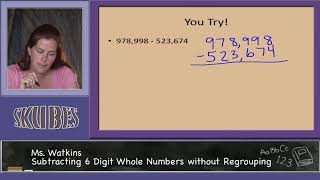By Skubes ed

Fluently add and subtract multi-digit whole numbers using the standard algorithm# Subtracting Fractions from a Whole Number

##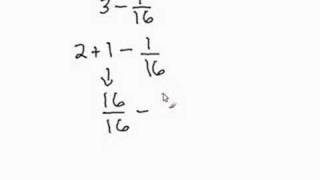By Davitily

In this short video students can learn how to subtract fractions from a whole number. The instructor uses computer software to demonstrate. Only one example is used in this video: 3-1/16.# Learn Fractions - Adding and subtracting fractions with whole and mixed numbers

##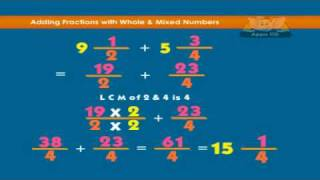By Appuseries

Learn Fractions - Adding and subtracting fractions with whole and mixed numbers ' a feature in the APPU Series.# Subtraction | Subtracting Whole Numbers | MathHelp.com

##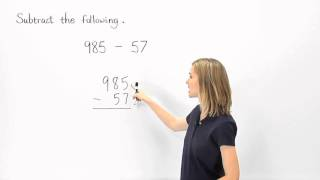By MathHelp.com

MathHelp.com makes learning Algebra easy with custom math courses with a teacher. Courses include Pre-Algebra, Algebra 1, Algebra 2, Introductory Algebra, Intermediate Algebra, and College Algebra. We also offer over 100 standardized test prep courses, such as COMPASS, ACCUPLACER, ASVAB, PRAXIS, and more.##By Mary Winter

Fluently add and subtract multi-digit whole numbers using the standard algorithm# Adding Fractions with Like Denominators

##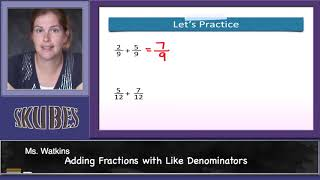By Skubes ed

Understand addition and subtraction of fractions as joining and separating parts referring to the same whole# [4.NBT.4-2.0] Add/Sub multi digit - Common Core Standard

##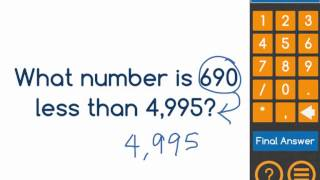By Freckle education

Fluently subtract multi-digit whole numbers using the standard algorithm.# [4.NF.3a-1.0] Intro to Fraction Addition - Common Core Standard - Word Problem

##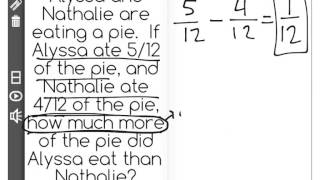By Freckle education

Understand addition and subtraction of fractions as joining and separating parts referring to the same whole# [4.NF.3a-1.0] Intro to Fraction Addition - Common Core Standard

##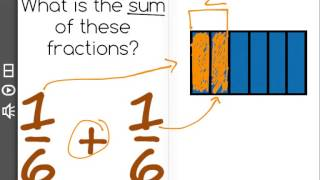By Freckle education

Understand addition and subtraction of fractions as joining and separating parts referring to the same whole# Subtracting Mixed Numbers With Regrouping

##By eHow

When subtracting mixed numbers with regrouping solve the equation of the fractions before working on the whole numbers. A math instructor explains how to create a matching denominator to subtract mixed numbers. He shows examples with paper and marker. Example included: 10 2/7 - 6 4/7.# Subtracting Mixed Numbers - YourTeacher.com - Pre Algebra Help

##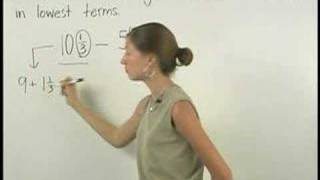By yourteachermathhelp

For a complete lesson on subtracting mixed numbers go to http://www.yourteacher.com - 1000+ online math lessons featuring a personal math teacher inside every lesson! In this lesson students learn to subtract mixed numbers by first subtracting the fractions then subtracting the whole numbers. For example to subtract 6 1/3 - 4 2/3 first subtract 1/3 ï¿½ 2/3. However notice that 1/3 ï¿½ 2/3 equals a negative fraction. In this situation the first fraction 6 1/3 can be rewritten as 5 + 1 1/3 or 5 + 4/3 or 5 4/3. Therefore the original problem 6 1/3 - 4 2/3 can be rewritten as 5 4/3 - 4 2/3. Now subtract the fractions 4/3 ï¿½ 2/3 to get 2/3 and subtract the whole numbers 5 ï¿½4 to get 1. So 5 4/3 - 4 2/3 = 1 2/3. Note that some of the problems in this lesson also require the student to find a common denominator for the fractions. For example 8 5/16 - 1 1/8.# Subtracting Fractions in a Word Problem

##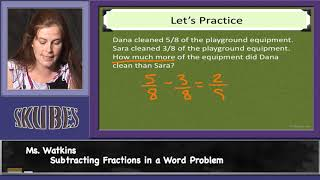By Skubes ed

Solve word problems involving addition and subtraction of fractions referring to the same whole and having like denominators# Multistep Fraction Problems

##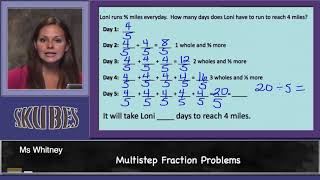By Skubes ed

Solve word problems involving addition and subtraction of fractions referring to the same whole and having like denominators# MULTIPLYING FRACTIONS WORD PROBLEMS

##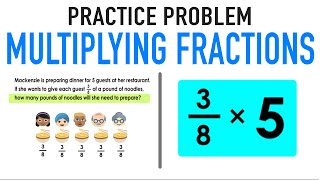By Mashup Math

Solve word problems involving addition and subtraction of fractions referring to the same whole and having like denominators# [4.NF.3a-1.0] Intro to Fraction Addition - Common Core Standard - Word Problem

##By Freckle education

Understand a fraction a/b with a greater than 1 as a sum of fractions 1/b. Understand addition and subtraction of fractions as joining and separating parts referring to the same whole.# [4.NF.3a-1.0] Intro to Fraction Addition - Common Core Standard

##By Freckle education

Understand a fraction a/b with a greater than 1 as a sum of fractions 1/b. Understand addition and subtraction of fractions as joining and separating parts referring to the same whole.# Dividing Integers | MathHelp.com

##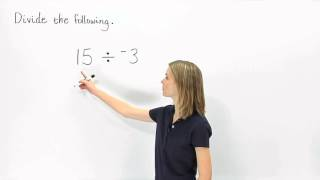By MathHelp.com

This lesson covers basic subtraction in the form of subtracting whole numbers. Students learn to subtract numbers with two or more digits, such as 985 - 47. The first step is to line up the numbers vertically so that the units digits are in the same column. Next, subtract the units digits, the tens digits, and the hundreds digits. When subtracting the units digits, notice that it is not possible to subtract 7 ones from 5 ones, so 1 ten must be borrowed from the tens column, leaving 7 tens and 15 ones. Now, subtracting the units digits, 15 - 7 = 8, subtracting the tens digits, 7 - 4 = 3, and subtracting the hundreds digits, 9 - 0 = 9. So 985 - 47 = 938. Note that the answer to a subtraction problem is called the difference, so the difference of 985 - 47 is 938.# [4.NF.3d-1.0] Word Problems: Addition - Common Core Standard

##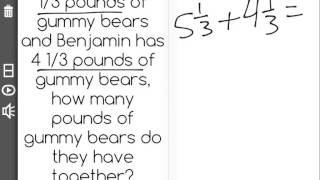By Freckle education

Solve word problems involving addition and subtraction of fractions referring to the same whole and having like denominators, e.g., by using visual fractions models and equations to represent to problem.# Adding and Subtracting Angles: 4.MD.7

##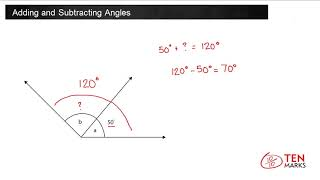By TenmarksAmazon

Recognize angle measure as additive. When an angle is decomposed into non-overlapping parts, the angle measure of the whole is the sum of the angle measures of the parts. Solve addition and subtraction problems to find unknown angles on a diagram in real world and mathematical problems##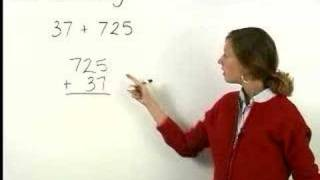By yourteachermathhelp